# Nodal Analysis in Electric Circuits (Example With Step by Step Analysis)

## Definition of Nodal Analysis

Nodal analysis is a method that provides a general procedure for analyzing circuits using node voltages as the circuit variables. Nodal Analysis is also called the Node-Voltage Method.
Some Features of Nodal Analysis are as

• Nodal Analysis is based on the application of the Kirchhoff’s Current Law (KCL).
• Having ‘n’ nodes there will be ‘n-1’ simultaneous equations to solve.
• Solving ‘n-1’ equations all the nodes voltages can be obtained.
• The number of non reference nodes is equal to the number of Nodal equations that can be obtained.

## Types of Nodes in Nodal Analysis

• Non Reference Node – It is a node which has a definite Node Voltage. e.g. Here Node 1 and Node 2 are the Non Reference nodes
• Reference Node – It is a node which acts a reference point to all the other node. It is also called the Datum Node.

### Types of Reference Nodes

1. Chassis Ground – This type of reference node acts a common node for more than one circuits.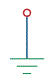2. Earth Ground – When earth potential is used as a reference in any circuit then this type of reference node is called Earth Ground.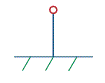## Solving of Circuit Using Nodal Analysis

#### Basic Steps Used in Nodal Analysis

1. Select a node as the reference node. Assign voltages V1, V2… Vn-1 to the remaining nodes. The voltages are referenced with respect to the reference node.
2. Apply KCL to each of the non reference nodes.
3. Use Ohm’s law to express the branch currents in terms of node voltages.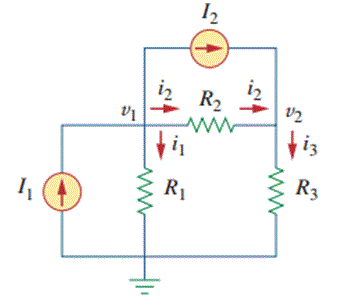Node Always assumes that current flows from a higher potential to a lower potential in resistor. Hence, current is expressed as follows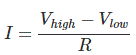IV. After the application of Ohm’s Law get the ‘n-1’ node equations in terms of node voltages and resistances.

V. Solve ‘n-1’ node equations for the values of node voltages and get the required node Voltages as result.

### Nodal Analysis with Current Sources

Nodal analysis with current sources is very easy and it is discussed with a example below.

Example: Calculate Node Voltages in following circuit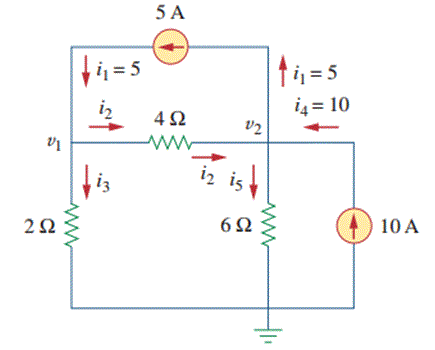In the following circuit we have 3 nodes from which one is reference node and other two are non reference nodes – Node 1 and Node 2.

Step I. Assign the nodes voltages as v1 and 2 and also mark the directions of branch currents with respect to the reference nodesStep II. Apply KCL to Nodes 1 and 2
KCL at Node 1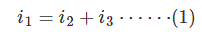KCL at Node 2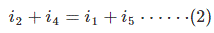Step III. Apply Ohm’s Law to KCL equations
Ohm’s law to KCL equation at Node 1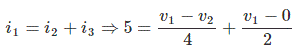Simplifying the above equation we get,• Now, Ohm’s Law to KCL equation at Node 2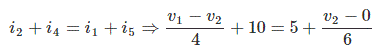Simplifying the above equation we get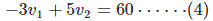Step IV. Now solve the equations 3 and 4 to get the values of v1 and v2 as,
Using elimination method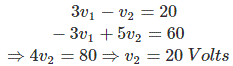And substituting value v2 = 20 Volts in equation (3) we get-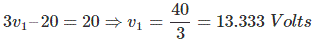Hence node voltages are as v1 = 13.33 Volts and v2 = 20 Volts.

## Nodal Analysis with Voltage Sources

Case I. If a voltage source is connected between the reference node and a non reference node, we simply set the voltage at the non-reference node equal to the voltage of the voltage source and its analysis can be done as we done with current sources. v1 = 10 Volts.

Case II. If the voltage source is between the two non reference nodes then it forms a supernode whose analysis is done as following

### Supernode Analysis

#### Definition of Super Node

Whenever a voltage source (Independent or Dependent) is connected between the two non reference nodes then these two nodes form a generalized node called the Super node. So, Super node can be regarded as a surface enclosing the voltage source and its two nodes.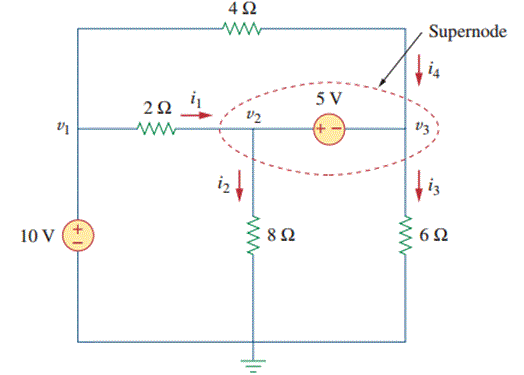In the above Figure 5V source is connected between two non reference nodes Node – 2 and Node – 3. So here Node – 2 and Node – 3 form the Super node.

#### Properties of Supernode

• Always the difference between the voltage of two non reference nodes is known at Supernode.
• A supernode has no voltage of its own
• A supernode requires application of both KCL and KVL to solve it.
• Any element can be connected in parallel with the voltage source forming the supernode.
• A Supernode satisfies the KCL as like a simple node.

#### How Solve Any Circuit Containing Supernode

Let’s take a example to understand how to solve circuit containing Supernode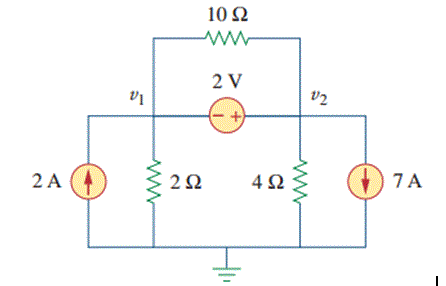Here 2V voltage source is connected between Node-1 and Node-2 and it forms a Supernode with a 10Ω resistor in parallel.
Note – Any element connected in parallel with the voltage source forming Super node doesn’t make any difference because v2– v1 = 2V always whatever may be the value of resistor. Thus 10 Ω can be removed and circuit is redrawn and applying KCL to the supernode as shown in figure gives,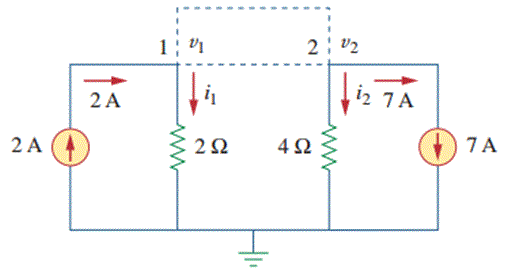Expressing and in terms of the node voltages.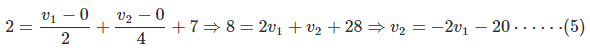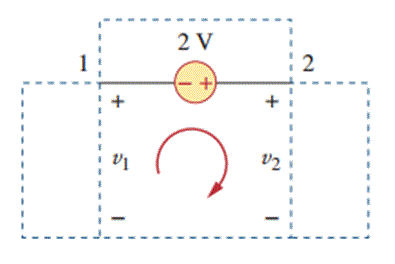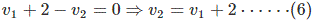From Equation 5 and 6 we can write as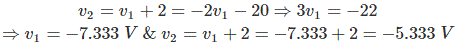Hence, v1 = – 7.333V and v2 = – 5.333V which is required answer.

Want To Learn Faster? 🎓
Get electrical articles delivered to your inbox every week.
No credit card required—it’s 100% free.